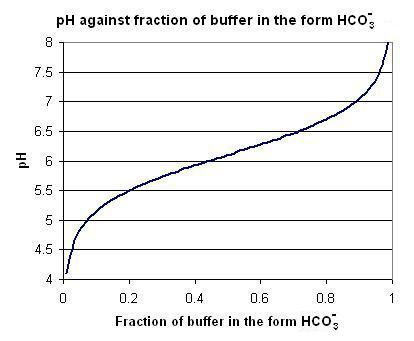#### You may also like### Very Old Man

Is the age of this very old man statistically believable?### bioNRICH

bioNRICH is the area of the stemNRICH site devoted to the mathematics underlying the study of the biological sciences, designed to help develop the mathematics required to get the most from your study of biology at A-level and university.### Catalyse That!

Can you work out how to produce the right amount of chemical in a temperature-dependent reaction?

# Blood Buffers

##### Age 16 to 18Challenge Level

In this question [$A$] means the concentration of the chemical $A$ at equilibrium.

For a balanced chemical equation, where $A, B, C$ and $D$ are chemicals in aqueous solution and $a, b, c, d$ are whole numbers,
$$aA + bB \rightleftharpoons cC + dD+ eH_2O$$
the law of mass action tells us that for a fixed temperature, there is a constant $K$ (called the equilibrium constant) such that
$$\frac{[C]^c[D]^d}{[A]^a[B]^b} = K$$
(note the absence of the solvent concentration $[H_2O]$ ).
In the blood, the carbonic-acid-bicarbonate buffer prevents large changes in the pH of the blood. Chemically, it consists of two reactions which are simultaneously in equilibrium

$$H^+ +HCO^-_3+H_2O\rightleftharpoons^{K_1} H_2CO_3+H_2O \rightleftharpoons^{K_2} 2H_2O+CO_2$$
Show that
$$pH =pK -\log \left(\frac{[CO_2]}{[HCO^-_3]}\right)\quad \mbox{where }K=\frac{1}{K_1K_2}$$

Think about this equation. It shows that the pH of the blood is dependent on the ratio of the concentrations of $CO_2$ and $HCO^-_3$. These are large in the blood, so small changes in the relative concentrations leads to very small changes in the pH of the blood. They act as a 'buffer' against pH change.

Now, make a new variable $x$ to be the fraction of the buffer in the form of $HCO^-_3$. Thus,
$$x = \frac{[HCO^-_3]}{[HCO^-_3]+[H_2CO_3]+[CO_2]}$$
Show that
$$pH = pK-\log\left(\frac{1}{x}-1 -K_1[H^+]\right)$$
By taking the value $pK=6.1$ and treating $K_1[H^+]$ as very small, reproduce the titration curveExtension:
1. Why is it numerically valid to ignore the $[H^+]$ term in the equation giving rise to the graph?
2. With the assumption that $K_1[H^+]=0$, use calculus to show that the second derivative of the pH is zero when $x=0.5$. Graphically, what does this correspond to?